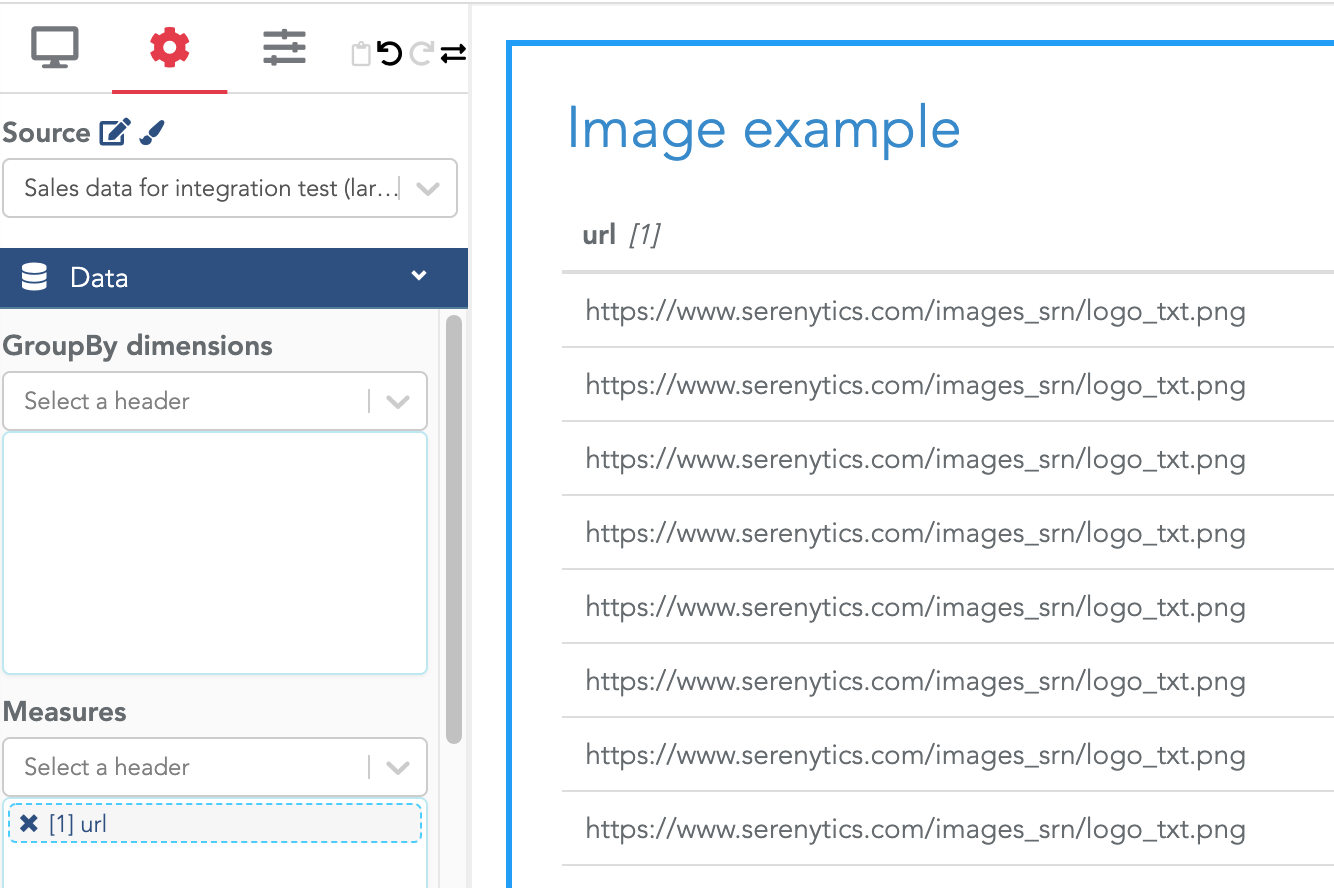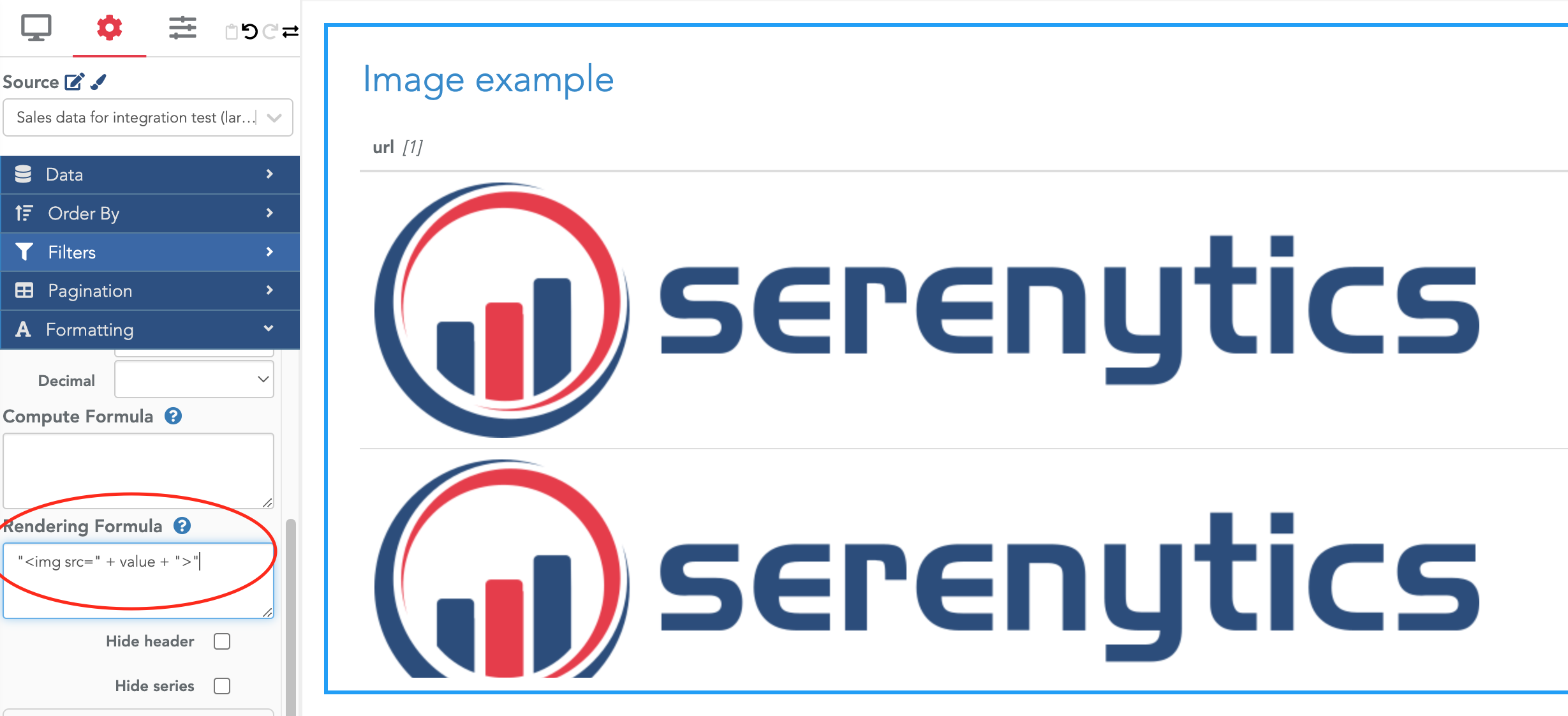# Show images in a tableConfigure the rendering formula

Let's say you have a column in a table that contains the URL of an image, for example: "https://www.serenytics.com/images_srn/logo_txt.png"

Of course, this is only an example and it would make more sense to have different URLs for each row.To show the actual image in the column instead of showing the URL string, you can use the fact that the rendering formula can be used to generate HTML code.

In the Rendering formula of you column, enter the formula : `"<img src=" + value + ">"`

In this formula, value contains the actual value of the cell (i.e. the URL). Note that you must copy/paste the full formula, including the double quotes:By default, it uses the image as it is (e.g. with its original size). You might have to customize the style of the image as explained in the next section.
Change the size and style of your image

To modify the design of your image (e.g. itw size), you can use CSS.

The first step is to modify your formula to specify a CSS class. You need to replace the previous formula with: `"<img class=myImgClass src=" + value + ">"`

Then, in the Advanced Style Editor, you need to customize your class, for example:

``````.myImgClass {
max-width: 150px;
}
``````

The full example is:×